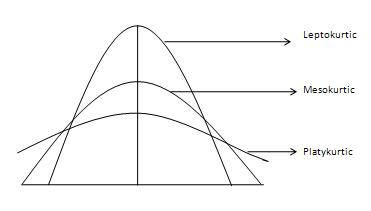# Statistics - Kurtosis

The degree of tailedness of a distribution is measured by kurtosis. It tells us the extent to which the distribution is more or less outlier-prone (heavier or light-tailed) than the normal distribution. Three different types of curves, courtesy of Investopedia, are shown as follows −It is difficult to discern different types of kurtosis from the density plots (left panel) because the tails are close to zero for all distributions. But differences in the tails are easy to see in the normal quantile-quantile plots (right panel).

The normal curve is called Mesokurtic curve. If the curve of a distribution is more outlier prone (or heavier-tailed) than a normal or mesokurtic curve then it is referred to as a Leptokurtic curve. If a curve is less outlier prone (or lighter-tailed) than a normal curve, it is called as a platykurtic curve. Kurtosis is measured by moments and is given by the following formula −

## Formula

${\beta_2 = \frac{\mu_4}{\mu_2}}$

Where −

• ${\mu_4 = \frac{\sum(x- \bar x)^4}{N}}$

The greater the value of \beta_2 the more peaked or leptokurtic the curve. A normal curve has a value of 3, a leptokurtic has \beta_2 greater than 3 and platykurtic has \beta_2 less then 3.

### Example

Problem Statement:

The data on daily wages of 45 workers of a factory are given. Compute \beta_1 and \beta_2 using moment about the mean. Comment on the results.

Wages(Rs.)Number of Workers
100-2001
120-2002
140-2006
160-20020
180-20011
200-2003
220-2002

Solution:

Wages
(Rs.)
Number of Workers
(f)
Mid-pt
m
m-${\frac{170}{20}}$
d
${fd}$${fd^2}$${fd^3}$${fd^4} 100-2001110-3-39-2781 120-2002130-2-48-1632 140-2006150-1-66-66 160-2002017000000 180-20011190111111111 200-200321026122448 220-2002230361854162 {N=45} {\sum fd = 10}$${\sum fd^2 = 64}$${\sum fd^3 = 40}$${\sum fd^4 = 330}$

Since the deviations have been taken from an assumed mean, hence we first calculate moments about arbitrary origin and then moments about mean. Moments about arbitrary origin '170'

${\mu_1^1= \frac{\sum fd}{N} \times i = \frac{10}{45} \times 20 = 4.44 \\[7pt] \mu_2^1= \frac{\sum fd^2}{N} \times i^2 = \frac{64}{45} \times 20^2 =568.88 \\[7pt] \mu_3^1= \frac{\sum fd^2}{N} \times i^3 = \frac{40}{45} \times 20^3 =7111.11 \\[7pt] \mu_4^1= \frac{\sum fd^4}{N} \times i^4 = \frac{330}{45} \times 20^4 =1173333.33 }$

${\mu_2 = \mu'_2 - (\mu'_1 )^2 = 568.88-(4.44)^2 = 549.16 \\[7pt] \mu_3 = \mu'_3 - 3(\mu'_1)(\mu'_2) + 2(\mu'_1)^3 \\[7pt] \, = 7111.11 - (4.44) (568.88)+ 2(4.44)^3 \\[7pt] \, = 7111.11 - 7577.48+175.05 = - 291.32 \\[7pt] \\[7pt] \mu_4= \mu'_4 - 4(\mu'_1)(\mu'_3) + 6 (\mu_1 )^2 (\mu'_2) -3(\mu'_1)^4 \\[7pt] \, = 1173333.33 - 4 (4.44)(7111.11)+6(4.44)^2 (568.88) - 3(4.44)^4 \\[7pt] \, = 1173333.33 - 126293.31+67288.03-1165.87 \\[7pt] \, = 1113162.18 }$

From the value of movement about mean, we can now calculate ${\beta_1}$ and ${\beta_2}$:

${\beta_1 = \mu^2_3 = \frac{(-291.32)^2}{(549.16)^3} = 0.00051 \\[7pt] \beta_2 = \frac{\mu_4}{(\mu_2)^2} = \frac{1113162.18}{(546.16)^2} = 3.69 }$

From the above calculations, it can be concluded that ${\beta_1}$, which measures skewness is almost zero, thereby indicating that the distribution is almost symmetrical. ${\beta_2}$ Which measures kurtosis, has a value greater than 3, thus implying that the distribution is leptokurtic.